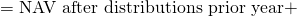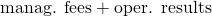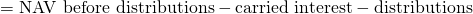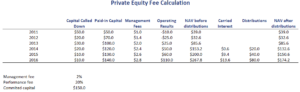# Private Equity Fee Calculation

Private Equity (PE) fee calculation is a topic investors should have a good understanding of. Fees are generally higher and harder to calculate than in the case of mutual funds. In particular, the fact that the general partners earning a performance fee or carried interest is something that is often new for most investors.

On this page, we discuss the discuss the 2 and 20 fee structure of private equity firms. We discuss the private equity management fee calculation and carried interest calculation. We finish with a private equity fee structure example that implements the approach in detail. The spreadsheet used is available at the bottom of the page.

## Private equity fund fee structure definition

Private equity fee calculation is best understood once we grasp the following concepts. In particular, we need to understand what capital called down, paid-in capital, NAV before distributions, carried interest, and NAV after distributions are.

Let’s discuss all of these concepts first. Capital called down is the capital that investors have transferred to the private equity firm during a given period. The paid-in capital is the cumulative capital called down. next, there is the NAV before distributions. NAV before distribution equalsThe NAV after distributions can then be calculated as followsFinally, there is the carried interest. The calculation is different the first year and in subsequent years. The first year is 20% multiplied by the NAV before distributions minus the committed capital. In subsequent years, it equals the increase in the NAV before distributions times 20%.

## Private equity fee structure example

The best way to illustrate all the necessary calculations is by using a table. The following table illustrates the calculation of PE fee calculation for a private equity funds. In particular, we calculate the total fees for a private equity fund after 6 years.## Summary

We discussed the calculation of private equity fees. Fees consist of a management fee and an incentive fee. While the private equity management fee calculation is straightforward, the calculation of the carried interest is less trivial. One of the reasons is that multiple distribution waterfall systems exist, so it is important to determine what type is used.